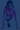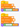Stay up to date with everything we do! Join the Mailing List!# Variable Introduction

Code Championship Puzzle - 2019-05-07

Difficulty: Level 1

## Can you solve this puzzle? What is the Answer?

Take a look at the code below and see if you can find the answer! What is the Answer that the computer will have at the end of these lines of code? When you think you have the answer. Click the "Show Answer" button!

In this puzzle, we are creating a variable and making changes to it. This puzzle introduces the concept of variables. If the Answer in the first section of code is "11", what is the answer for the second section of code?If you liked this puzzle, you should check out the other things we do!